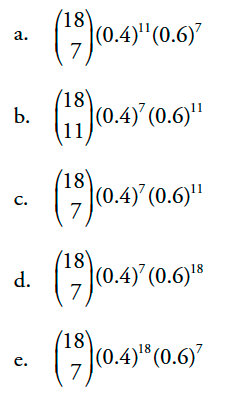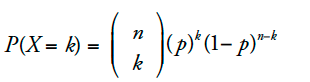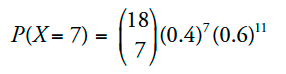# 【每日一题】AP 统计学 20180129

AP Statistics

Eighteen trials of a binomial random variable X are conducted. If the probability of success for any one trial is 0.4, write the mathematical expression you would need to evaluate to find P(X = 7). Do not evaluate.Binomial distribution二项分布即重复多次独立的试验，在每次试验中只有成功或失败的结果（也可以是类似的其他两种不相容的结果）。